# HC Verma Solutions Class 11 Chapter 7 Circular Motion

HC Verma Solutions class 11 Chapter 7 Circular Motion is provided here with a fresh approach and extensive coverage of various topics related to the vertical and horizontal component of velocity and angular velocity etc. HCV Vol 1 and Vol 2 books are highly recommended and will help students develop better skills and prepare efficiently for IIT JEE as well as other competitive exams. However, in this chapter;

• We will be seeing questions on the distance between Earth and Moon is given and students have to find the acceleration.
• We will be solving questions related to types of acceleration such as radial acceleration, tangential acceleration and magnitude of the acceleration
• We will deal with questions on the angle of banking and deal with problems related to the centripetal force of the electron.

To further help students understand the concept clearly, the HCV Solutions Vol 1 provided here have been developed by experts. These solutions contain accurate answers to all the questions asked in chapter 7 of the HC Verma book for class 11. Practising the problems given in solutions will definitely help students clear their doubts and perform better in the exams.

Students will get to know about topics like;

1. Angular Variables
2. Unit Vectors along the Radius and the Tangent
3. Acceleration in Circular Motion
4. Dynamics of Circular Motion
5. Circular Turnings and Banking of Roads
6. Centrifugal Force
7. Effect of Earth’s Rotation on Apparent Weight

## Class 11 Important Questions In Chapter 7

1. A car moves on a horizontal road with a uniform velocity. Can the car be accelerated to gain some speed without increasing the petrol flow rate into the engine?
2. An eagle while hunting for its prey sees its target on one side and it takes a sharp left turn. Where does the eagle get the centripetal force from?
3. A small piece of metal with mass m is tied to a thread measuring length 1. The metal is rotated in a circular motion and the other end of the string is tied at the centre. The speed of the metal body is v. What will happen to the metal piece if the thread cuts in between. (a) move away from the centre (b) move towards the centre (c) comes to a halt and stops (d) be thrown along a tangent
4. Suppose one day the earth stops rotating. What will happen to the apparent value of g on its surface?               (a) remain the same everywhere (b) decrease everywhere (c) increase everywhere (d) increase at some places and remain the same at some places.
5. When an object is moving along a curved path what quantities will remain constant during the motion?          (a) the magnitude of acceleration (b) velocity (c) acceleration (d) speed.

## HC Verma Solutions Vol 1 Circular Motion Chapter 7

Question 1: Find the acceleration of the moon with respect to the earth from the following data: Distance between the earth and the moon = 385 × 105 km and the time taken by the moon to complete one revolution around the earth = 273 days.

Solution:

Distance between the earth and the moon = Radius of Revolution = R = 385 × 105 m

Angular frequency, ω = 2πf = 2π/T

Time taken by moon to complete one revolution = T = 273 days = 273 × 24 × 60 × 60 secs = 23587200 secs

Acceleration of the moon = ω2R = (2π/T) 2R = 4π2R/T2

Substituting the values, we have

Acceleration of the moon = 2.73 × 10-7 m/s2

Question 2: Find the acceleration of a particle placed on the surface of the earth at the equator due to earth’s rotation. The diameter of earth = 12800 km and it takes 24 hours for the earth to complete one revolution about its axis.

Solution:

Radius of earth = R = half of diameter = Diameter of earth/2 = 12800/2 km = 6400 km = 6.4 × 106 m

Time taken for one rotation = T = 24 hours = 24 × 60 ×60 sec = 86400 secs

Angular velocity of the particle, ω = 2π/T

Therefore, acceleration of the particle = Rω2

Substituting the values, we have

acceleration of the particle = 0.0338 m/s2

Question 3: A particle moves in a circle of radius 1.0 cm at a speed given by u = 2.0 t where v is in cm/s and t in seconds. (a) Find the radial acceleration of the particle at t= 1 s. (b) Find the tangential acceleration at t = 1 s. (c) Find the magnitude of the acceleration at t = 1 s.

Solution:

V = 2t and Radius = r = 1.0 cm

(a) At t = 1s

Velocity of the particle, u = 2.0 t = 2.0×1 = 2.0 cm/s

Radial acceleration of the particle at t = 1 is v2/r = (2.0) 2/1 = 4 cm/s2

(b)

We know, a = dv/dt = d/dt(2t) = 2 cm/s2

Tangential acceleration at t=1 is 2 cm/s2

(c) Magnitude of acceleration at t = 1sec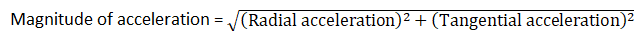= √20 cm/s2

Question 4: A scooter weighing 150 kg together with its rider moving at 36 km/hr is to take a turn of radius 30 m. What horizontal force on the scooter is needed to make the turn possible?

Solution:

Mass of the scooter = M = 150 kg

Velocity = v = 36 km/hr = 36 × 5/18 m/s = 10 m/s

and Radius = r = 30 m

Horizontal force required = centripetal force = Mv2/r

=150×10×10/30

= 500 N

Question 5: If the horizontal force needed for the turn in the previous problem is to be supplied by the normal force by the road, what should be the proper angle of banking?

Solution: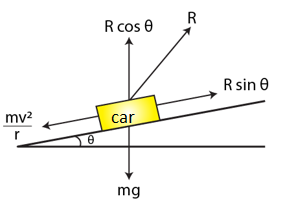Velocity of the car = v = 10m/s

Consider,

mass of the car=m, acceleration due to gravity = g, Angle of banking = θ and Normal reaction = R

From the diagram,

R cosθ = mg

R sinθ = mv2/r

Now,

Tanθ = v2/rg

or θ = tan-1(v2/rg)

or θ = tan-1(10×10/30)

or θ = tan-1(1/3): Angle of banking

Question 6: A park has a radius of 10 m. If a vehicle goes round it at an average speed of 18 km/hr, what should be the proper angle of banking?

Solution:

Given:

Average speed = v = 18km/hr or 5 m/s

Radius of park = r = 10 m

Acceleration due to gravity = g = 10m/s2

We know, angle of banking, θ = tan-1(v2/rg)

= tan-1(1/4)

Question 7: If the road of the previous problem is horizontal (no banking), what should be the minimum friction coefficient so that a scooter going at 18 km/hr does not skid?

Solution:

The road is horizontal (no banking)

mv2/R = μN and N = mg

So, mv2/R = μmg

=> 25/10 = μg

=> μ = 25/100 = 0.25

Question 8: A circular road of radius 50 m has the angle of banking equal to 30o. At what speed should a vehicle go on this road 80 that the friction is not used?

Solution:

We know that, tanθ = v2/rg

Radius of the circular path = r = 50 m

Acceleration due to gravity = g = 10m/s2

Angle of banking = θ = 30°

=> tan 30° = v2/(50×10)

=> v = 17 m/s

Vehicle should go with a speed of 17 m/s.

Question 9: In the Bohr model of hydrogen atom, the electron is treated as a particle going in a circle with the centre at the proton. The proton itself is assumed to be fixed in an inertial frame. The centripetal force is provided by the Coulomb attraction. In the ground state, the electron goes round the proton in a circle of radius 5.3 × 10-11 m. Find the speed of the electron in the ground state. Mass of the electron = 9.1 × 10-31 kg and charge of the electron = 1.6 × 10-19 C.

Solution:

Centripetal force is provided by coulomb attraction.

Mass of electron, m = 9.1 × 10-31 kg

Radius of the circular path, r = 5.3 × 10-11 m

k = 1/(4πε0) = 9×109

q1 = charge on an electron= q2 = charge on the proton = q = 1.6 × 10-19 C

We know, v2 = kq2/mr

=> v2 = [9×109×1.6×10-19×1.6×10-19] / [9.31×10-31×5.3×10-11]

or v = 2.2 × 106 m/s (approx)

Which is the speed of the electron in ground state.

Question 10: A stone is fastened to one end of a string and is whirled in a vertical circle of radius R. Find the minimum speed the stone can have at the highest point of the circle.

Solution:

At the highest point of a vertical circle.

mv2/R = mg

=> v = √Rg

Question 11: A ceiling fan has a diameter (of the circle through the outer edges of the three blades) of 120 cm and rpm 1500 at full speed. Consider a particle of mass 1 g sticking at the outer end of a blade. How much force does it experience when the fan runs at full speed? Who exerts this force on the particle? How much force does the particle exert on the blade along its surface?

Solution:

Diameter = d = 120 cm = 1.2 m

Radius = r = d/2 = 0.6 m

Frequency = f = 1500 rpm = 1500/60 rps = 25 s-1

Now,

Angular frequency, ω = 2πf = 157.14 rad s-1

[putting value of f]

Again,

Mass of the particle, m = 1g =0.001 kg

Force on the particle on the blade = mrω2

= 0.001 × 0.6 × 157.14 ×157.14

= 14.81 N

The fan runs at the full speed in circular path. This exerts the force on the particle.

Force exerted by the particle on the blade = Force exerted on the particle = 14.81 N

Question 12: A mosquito is sitting on an L.P. record disc rotating on a turn table at 33_1/3 revolutions per minute. The distance of the mosquito from the center of the turn table is 10 cm. Show that the friction coefficient between the record and the mosquito is greater than it π2/81. Take g= 10 m/s2.

Solution:

A mosquito is sitting on an L.P. record disc rotating on a turn table at 33_1/3 revolutions per minute. (given)

say, n = 33_1/3 rpm = 100/(3×60) rps

we know, ω = 2πn = 10π/9 rad/sec

Also, we are given with

radius = r = 10 cm or 0.1 m

and g = 10 m/sec2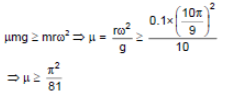Question 13: A simple pendulum is suspended from the ceiling of a car taking a turn of radius 10 m at a speed of 36 km/h. Find the angle made by the string of the pendulum with the vertical if this angle does not change during the turn. Take g = 10 m/s2.

Solution:

Radius = r = 10 m, speed = v = 36 km/h and g = 10 m/s2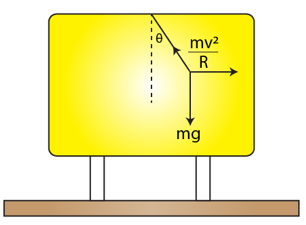The speed of the car is not changing. So, there is no force acting in the tangential direction of motion.

From the figure:

T sin θ = mv2/r ….(1)

and T cos θ = mg ….(2)

=> tan θ = v2/rg

or θ = tan-1(v2/rg)

After substituting and solving the above equation, we have

θ = 45o

Question 14: The bob of a simple pendulum of length 1 m has mass loo g and a speed of 1.4 m/s at the lowest point in its path. Find the tension in the string at this instant.

Solution:

A simple pendulum always constitutes some velocity at the lowest point. This proves that there exists a non-zero central force in the system. This central force will contribute to increase tension.At the lowest point:

T = mg + v2/r

Here m = 100g or 1/10 kg, r = 1m and v = 1.4 m/s

T = 1/10 x 9.8 x (1.4) 2/10 = 1.2 N

Question 15: Suppose the bob of the previous problem has a speed of 1.4 m/s when the string makes an angle of 0.20 radian with the vertical. Find the tension at this instant. You can use ≈ cosθ ≈ 1 – θ2/2 and sinθ ≈ θ, for small θ.

Solution: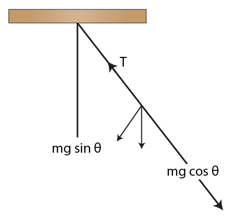The bob has a velocity 1.4 m/s, when the string makes an angle of 0.2 radian.

Here m = 100g or 0.1 kg and r = 1m and v = 1.4 m/s

From figure,

T – mg cos θ = mv2/r

=> T = 0.196 + 9.8(1-0.04/2)

[cosθ = 1 – θ2/2 for small θ]

=> T = 0.196 + (0.98)(0.98)

= 1.16N (approx)

Question 16: Suppose the amplitude of a simple pendulum having a bob of mass m is θo. Find the tension in the string when the bob is at its extreme position.

Solution:

When a simple pendulum reaches the extreme position, velocity of the pendulum is zero.

=> no centrifugal force.

=> T = mg cos θo

Question 17: A person stands on a spring balance at the equator. (a) By what fraction is the balance reading less than his true weight? (b) If the speed of earth’s rotation is increased by such an amount that the balance reading is half the true weight, what will be the length of the day in this case?

Solution:

(a) As person is standing at one position, he is in an equilibrium with the reaction force.

Net force on the spring balance:

R = mg – mω2r

The actual weight of the body,

W = mg

So, the decrement will be,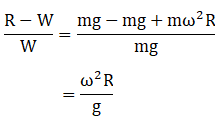and, Angular speed of earth, ω = 2π/(24×602) Hz

So, it will decrease at a rate,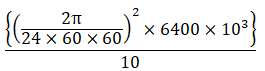= 3.5 × 10-3

(b) When the balance reading is half the true weight

mg – mω2R = 1/2 mg

(1/2) g = ω2 R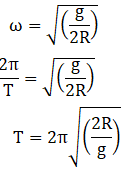= 7105 sec or 2 hrs(approx)

Question 18: A turn of radius 20 in is banked for the vehicles going at a speed of 36 km/h. If the coefficient of static friction between the road and the tyre is 0.4, what are the possible speeds of a vehicle so that it neither slips down nor skids up?

Solution: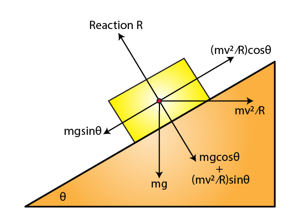Let θ be the path has an inclination. There is no movement in vertical direction. So,

R = mg cos θ + mv2/R sinθ

If the vehicle is not to be slide up then the friction must reinforce the overall force. Condition must be

μR = mg sinθ – mv2/R cos θ

From above equations, we have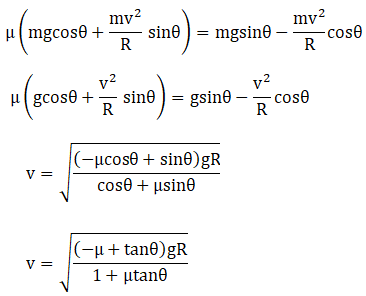Find tanθ:

tanθ = v2/Rg

Putting, v = (36×1000)/(60×60), R = 20 and g = 10, we have

tanθ = 0.5

=> v = 14.7 km/h

The condition for not to skid down will be

μR = mv2/R cosθ – mg sinθ

Solving above equation, we get

v = 54 km/h

The possible speeds are between 14.7 km/h and 54 km/h.

Question 19: A motorcycle has to move with a constant speed on an over-bridge which is in the form of a circular arc of radius R and has a total length L. Suppose the motorcycle starts from the highest point. (a) What can its maximum velocity be for which the contact with the road is not broken at the highest point? (b) If the motorcycle goes at speed 1/√2 times the maximum found in part (a), where will it lose the contact with the road? (c) What maximum uniform speed can it maintain on the bridge if it does not lose contact anywhere on the bridge?

Solution:

Let us consider, L be the total length of the over bridge and R be the radius of the bridge.

(a) At the highest point

mg = mv2/R

or v = √(Rg)

(b) Distance π R/3 along the bridge from the highest point,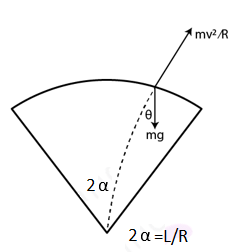The condition for just flying off will be,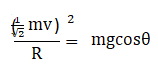or cos θ = 1/2

or θ = π/3

we can also write, θ = x/R

or x = πR/3, is the distance where it lose the contact when it moves with 1/√2 times the maximum.

(c) The chances of losing contact is maximum at the end of the bridge for which α = L/2R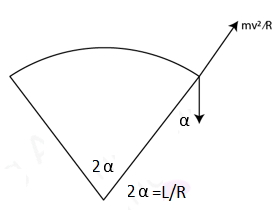=> mg cos α = mv2/R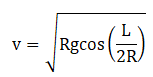Question 20: A car goes on a horizontal circular road of radius R, the speed increasing at a constant dv/dt=a. The friction coefficient between the road and the tyre is μ. Find the speed at which the car will skid.

Solution: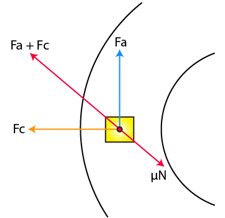The body is experiencing a force in the tangential direction as there exists some an acceleration along this direction.

friction force = F = μN

As the path is circular the body will also experience centrifugal force in the radial direction.

Fc = mv2/R

As there is no motion in vertical direction, normal reaction force will be mg

N = mg

Magnitude of the tangential force:

Fa = m dv/dt

=> Fa = ma

The car will skid when the friction will not be enough to reinforce Fa + Fc.

Condition for just skidding: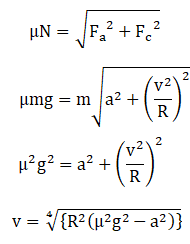Question 21: A block of mass m is kept on a horizontal ruler. The friction coefficient between the ruler and the block is μ. The ruler is fixed at one end and the block is at a distance L from the fixed end. The ruler is rotated about the fixed end in the horizontal plane through the fixed end. (a) What can the maximum angular speed be for which the block does not slip? (b) If the angular speed of the ruler is uniformly increased from zero at an angular acceleration a, at what angular speed will the block slip?

Solution: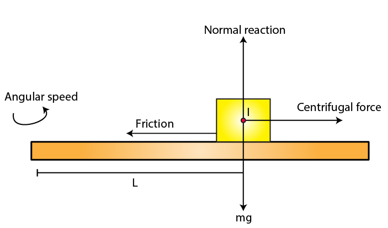(a)

When the system rotates the block will experience centrifugal force which will be balanced by friction.

Normal reaction will be balance the force of gravitation.

N = mg

FC = f

=> mω2 L = μ mg

or ω = √(μg)/L

(b)

When there comes some amount of acceleration, friction starts working in the opposite direction of motion.

Radial acceleration will be due to centrifugal force.

aR = ω2 L

and tangential acceleration, aT = dv/dt

= d/dt (Lω)

= L d/dt (ω)

=> aT = Lα

When friction reaches its maximum value, it will start slipping.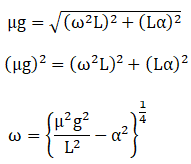Question 22: A track consists of two circular parts ABC and CDE of equal radius 100 m and joined smoothly as shown in figure (below). Each part subtends a right angle at its center. A cycle weighing 100 kg together with the rider travels at a constant speed of 18 km/h on the track. (a) Find the normal contact force by the road on the cycle when it is at B and at D. (b) Find the force of friction exerted by the track on the Lyres when the cycle is at B, C and D. (c) Find the normal force between the road and the cycle just before and just after the cycle crosses C. (d) What should be the minimum friction coefficient between the road and the tyre, which will ensure that the cyclist can move with constant speed? Take g = 10 m/s2.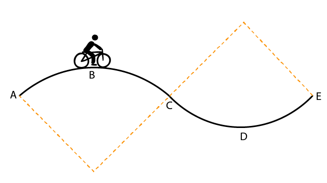Solution:

Radius of curve = R = 100 m

Weight = m = 100 kg

Velocity = v = 18km/h or 5m/sec

and g = 10 m/s2.

(a) At point B

mg – mv2/R = N

on substituting the values.

=> N = 975N

(b) At points B and D, the cycle has no tendency to slide.

So at these points friction of force is zero.

At Point C,

mg sin θ = F

=> F = 1000 x 1/√2 = 707 N

(c)

Before point C: mg cosθ – N = mv2/R

=> N = mg cosθ – mv2/R = 707 – 25 = 683N

and

N – mg cos θ = mv2/R

=> N = mv2/R + mg cos θ = 25 + 707 = 732N

(d) Consider a point just before C, where N is minimum.

μN = mg sinθ

=> μ x 682 = 707

=> μ = 1.037

Question 23: In a children’s park a heavy rod is pivoted at the center and is made to rotate about the pivot so that the rod always remains horizontal. Two kids hold the rod near the ends and thus rotate with the rod (figure below). Let the mass of each kid be 15 kg, the distance between the points of the rod where the two kids hold it be 30 m and suppose that the rod rotates at the rate of 20 revolutions per minute. Find the force of friction exerted by the rod on one of the kids.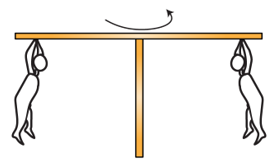Solution:

Radius= R = diameter/2 = 3/2 = 1.5 m

where R is the distance from the centre to one of the kids

Here, ω = 2πR = 2π/3

m = 15 kg (given)

Now, the frictional force on one of the kids = F = mRω2

= 15 x 1.5 x (2π/3) 2

= 10 π2

Question 24: A hemispherical bowl of radius R is rotated about its axis of symmetry which is kept vertical. A small block is kept in the bowl at a position where the radius makes an angle θ with the vertical. The block rotates with the bowl without any slipping. The friction coefficient between the block and the bowl surface is μ, Find the range of the angular speed for which the block will not slip.

Solution: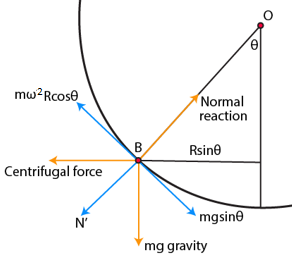Here gravity force and the centrifugal force are used.

Normal reaction force is balanced as,

N = mg cosθ + mω2 R sin^2θ

Here two cases of friction aries, one is along mgsinθ and the another in opposite direction as friction is a variable.

When friction is along mg sinθ

f = mω2 R cosθ sinθ – mg sinθ

when friction is at its maximum limit, we have

f = μN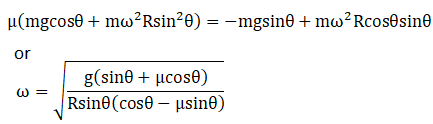…..(1)

When Friction is in the opposite direction of mgsinθ

f = mg sinθ – mω2 R cosθ sinθ

when friction is at its maximum limit, we have

f = μN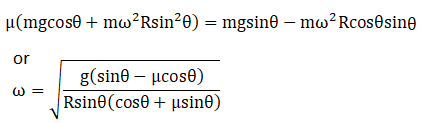…..(2)

The angular speed is lies in the range from (2) to (1) then the block will not slip.

Question 25: A particle is projected with a speed u at an angle θ with the horizontal. Consider a small part of its path near the highest position and take it approximately to be a circular arc. What is the radius of this circle? This radius is called the radius of curvature of the curve at the point.

Solution: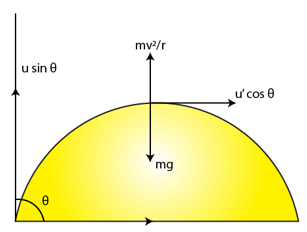A particle is projected with a speed u at an angle θ with the horizontal.

Vertical component of velocity is zero at the highest point.

consider at any point velocity is u cosθ

Centripetal force = mu2 cos2 (θ/2)

So, the radius of the circle at highest point is

mg = mv2/r

=> r = (u2 cos2θ)/g

Question 26: What is the radius of curvature of the parabola traced out by the projectile in the previous problem at a point where the particle velocity makes an angle θ/2 with the horizontal?

Solution: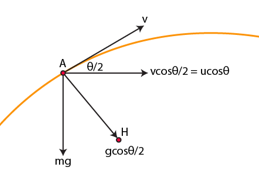Here g is in downwards direction. Hence, some component to g will act as central force which will be changing at each point depending upon θ.

Horizontal component of velocity will not change due to absence of force.

vH = u cosθ

or

vH = v cos(θ/2)

or v = ucosθ/cos(θ/2)

We know, central force = Fc = mv2/R

=> m g cos(θ/2) = mv2/R

=> R = (mu2 cos2θ)/(gcos3(θ/2))

Question 27: A block of mass m moves on a horizontal circle against the wall of a cylindrical room of radius R. The floor of the room on which the block moves is smooth but the friction coefficient between the wall and the block is μ. The block is given an initial speed u0. As a function of the speed u write (a) or the normal force by the wall on the block, (b) the frictional force by the wall and (c) the tangential acceleration of the block. (d) Integrate the tangential acceleration (du/dt = u . du/ds) to obtain the speed of the block after one revolution.

Solution:

(a) When normal reaction will be balancing the centrifugal force.

Fc = mv2/R

and normal reaction, N = mv2/R

(b) Friction force is at maximum as the body is moving,

Force of friction = f = μN = μmv2/R

(c)

The force changing the velocity will be friction.

f = μmv2/R

mass x acceleration = μmv2/R

or acceleration = -μv2/R

(d) dv/dt = v dv/dv

=> v(dv/ds) = -μv2/R

=> dv/v = -μ/R ds

for one complete revolution, s = 2πR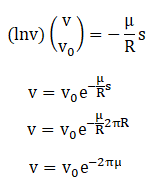Question 28: A table with smooth horizontal surface is fixed in a cabin that rotates with a uniform angular velocity ω in a circular path of radius R (figure below). A smooth groove AB of length L (<< R) is made on the surface of the table. The groove makes an angle θ with the radius OA of the circle in which the cabin rotates. A small particle is kept at the point A in the groove and is released to move along AB. Find the time taken by the particle to reach the point B.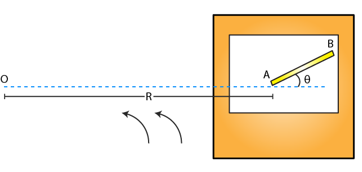Solution:

The cabin rotates with angular velocity ω and radius R.

The particle experience a force mRω2.

The component of mRω2 along groove provides the required force to the particle to move along AB.

mRω2 cosθ = ma

=> a = Rω2 cosθ

If length of groove be L then

L = ut + 1/2 at2

L = 1/2 R ω2 cosθ t2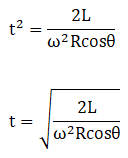Question 29: A car moving at a speed of 36 km/hr is taking a turn on a circular road of radius 50 m. A small wooden plate is kept on the seat with its plane perpendicular to the radius of the circular road (figure below). A small block of mass loo g is kept on the seat which rests against the plate. The friction coefficient between the block and the plate is μ = 058. (a) Find the normal contact force exerted by the plate on the block. (b) The plate is slowly turned so that the angle between the normal to the plate and the radius of the road slowly increases. Find the angle at which the block will just start sliding on the plate.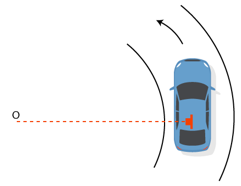Solution:

(a) The speed in MKS = v = 10m/s

The normal force will be balancing the centrifugal force.

Fc = N

N = mv2/R

N = 0.2 N

(b) The plate is turned so the angle between normal t the plate and the radius of the road slowly increases.

N = mv2/R cosθ …(1)

μN = mv2/R sinθ …2)

Solving (1) and (2), we have

μ = tanθ

μ = friction coefficient between plate and body = 0.58 (given)

or θ = tan-1 (0.58) = 30o

Question 30: A table with smooth horizontal surface is placed in a cabin which moves in a circle of a large radius R (figure below). A smooth pulley of small radius is fastened to the table. Two masses m and 2m placed on the table are connected through a string going over the pulley. Initially the masses are held by a person with the strings along the outward radius and then the system is released from rest (with respect to the cabin). Find the magnitude of the initial acceleration of the masses as seen from the cabin and the tension in the string.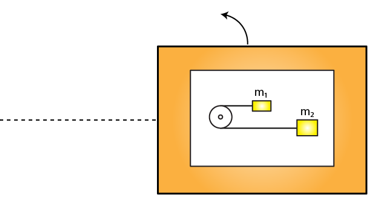Solution: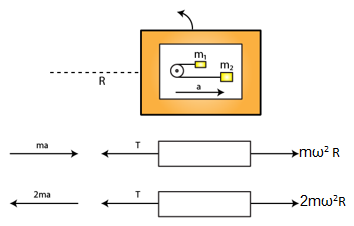From the free body diagrams,

T – ma – mω2 R = 0 …..(1)

T + 2ma – 2mω2R = 0 ….(2)

on subtracting (2) from (1)

=> 3 ma = mω2 R

=> a = mω2R/3

(1)=> T = 4/3 mω2 R